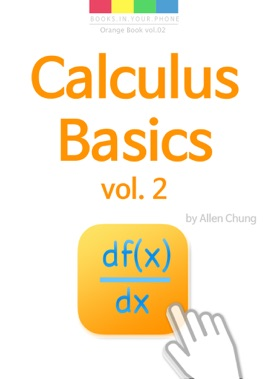• 4,49 €

## Descrizione dell’editore

This book is the second volume of Calculus Basics, which is composed of The Limits, The Differential Calculus, and The Integral Calculus. And it is intended for those who try to understand the basics of calculus or for the students preparing for the AP calculus test.

In the first volume, you learn the following topics:

▪︎ Definitions of Functions

▪︎ Algebraic and Transcendental Functions

▪︎ Definitions of Limits

▪︎ Theorems on Limits

▪︎ Evaluations of Limits

▪︎ Continuity of Functions

▪︎ Infinite Sequence

▪︎ Infinite Series

In the second volume, you learn the following topics:

▪︎ Definitions of Differentiation

▪︎ Derivatives

▪︎ Rules of Differentiation

▪︎ Analysis of Function Graphs

▪︎ Applications of Differential Calculus

In the third volume, you learn the following topics:

▪︎ Definitions of Integral

▪︎ Antidifferentiation

▪︎ Definite Integrals

▪︎ Fundamental Theorem of Calculus

▪︎ Rules of Antidifferentiation

▪︎ Applications of Integral Calculus

▪︎ Introduction to Differential Equations

▪︎ Infinite Series and Power Series

* This volume will be published soon.